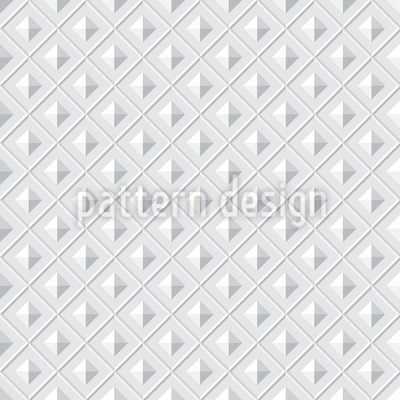••••# Squares From Above Seamless Vector Pattern Design

Keywords
Above, 3d, Square, Geometric, Bird's Eye View, Monochrome, Pyramid, Three-dimensional, Geometric Pattern, Geometrical Squares From Above design, Squares From Above vector, Squares From Above pattern, Squares From Above all over print, Squares From Above illustration, Squares From Above ornament, Squares From Above pattern design, Squares From Above artwork, Squares From Above vector art, Squares From Above background pattern, Squares From Above textile, Squares From Above seamless, Squares From Above repeating pattern, Squares From Above surface
Designer Edwin Candra Yani ID #6348
Description Great geometric design with pyramides and squaren from a bird's eye view.
Prices